This book is archived and will be removed July 6, 2022. Please use the updated version.

Interference

# 20 Mathematics of Interference

### Learning Objectives

By the end of this section, you will be able to:

• Determine the angles for bright and dark fringes for double slit interference
• Calculate the positions of bright fringes on a screen

(Figure)(a) shows how to determine the path length differencefor waves traveling from two slits to a common point on a screen. If the screen is a large distance away compared with the distance between the slits, then the angle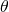between the path and a line from the slits to the screen [part (b)] is nearly the same for each path. In other words,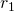and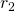are essentially parallel. The lengths ofanddiffer by, as indicated by the two dashed lines in the figure. Simple trigonometry shows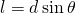where d is the distance between the slits. Combining this result with (Figure), we obtain constructive interference for a double slit when the path length difference is an integral multiple of the wavelength, or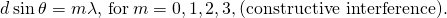Similarly, to obtain destructive interference for a double slit, the path length difference must be a half-integral multiple of the wavelength, or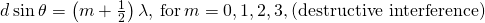where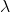is the wavelength of the light, d is the distance between slits, andis the angle from the original direction of the beam as discussed above. We call m the order of the interference. For example,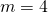is fourth-order interference.

(a) To reach P, the light waves from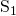and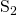must travel different distances. (b) The path difference between the two rays is.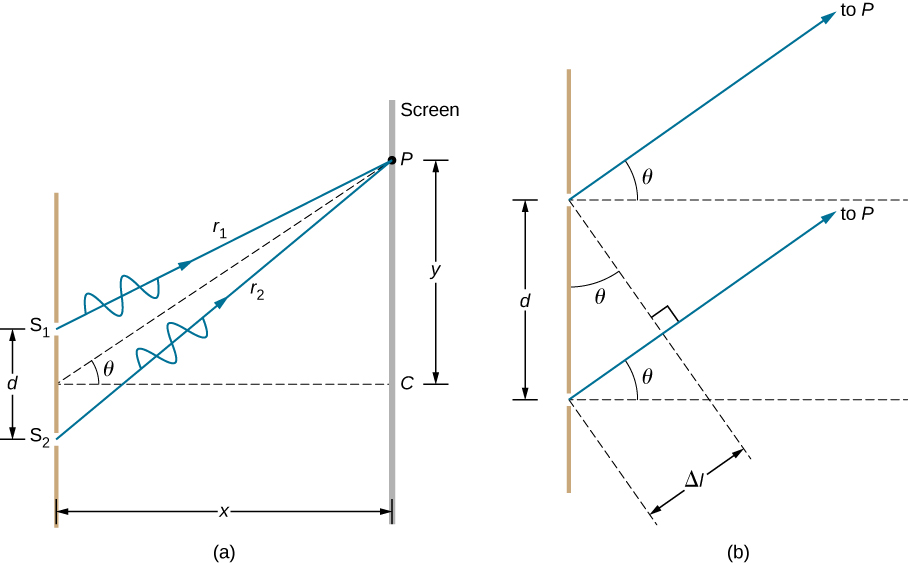The equations for double-slit interference imply that a series of bright and dark lines are formed. For vertical slits, the light spreads out horizontally on either side of the incident beam into a pattern called interference fringes ((Figure)). The closer the slits are, the more the bright fringes spread apart. We can see this by examining the equation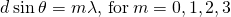. For fixedand m, the smaller d is, the largermust be, since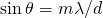. This is consistent with our contention that wave effects are most noticeable when the object the wave encounters (here, slits a distance d apart) is small. Small d gives large, hence, a large effect.

Referring back to part (a) of the figure,is typically small enough that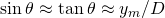, where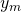is the distance from the central maximum to the mth bright fringe and D is the distance between the slit and the screen. (Figure) may then be written as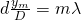or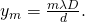The interference pattern for a double slit has an intensity that falls off with angle. The image shows multiple bright and dark lines, or fringes, formed by light passing through a double slit.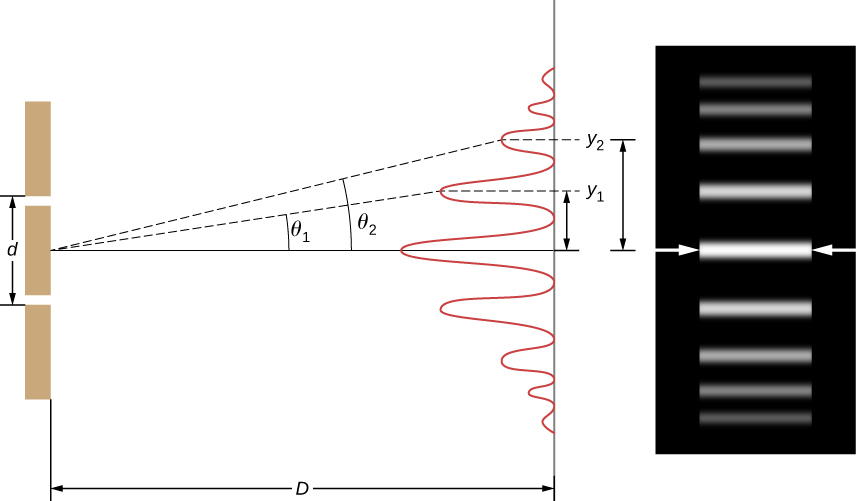Finding a Wavelength from an Interference Pattern Suppose you pass light from a He-Ne laser through two slits separated by 0.0100 mm and find that the third bright line on a screen is formed at an angle of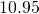relative to the incident beam. What is the wavelength of the light?

Strategy The phenomenon is two-slit interference as illustrated in (Figure) and the third bright line is due to third-order constructive interference, which means that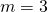. We are given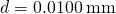and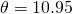. The wavelength can thus be found using the equation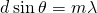for constructive interference.

Solution Solvingfor the wavelengthgives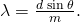Substituting known values yields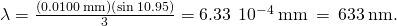Significance To three digits, this is the wavelength of light emitted by the common He-Ne laser. Not by coincidence, this red color is similar to that emitted by neon lights. More important, however, is the fact that interference patterns can be used to measure wavelength. Young did this for visible wavelengths. This analytical techinque is still widely used to measure electromagnetic spectra. For a given order, the angle for constructive interference increases with, so that spectra (measurements of intensity versus wavelength) can be obtained.

Calculating the Highest Order Possible Interference patterns do not have an infinite number of lines, since there is a limit to how big m can be. What is the highest-order constructive interference possible with the system described in the preceding example?

Strategy The equation(for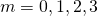) describes constructive interference from two slits. For fixed values of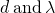, the larger m is, the larger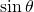is. However, the maximum value thatcan have is 1, for an angle of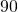. (Larger angles imply that light goes backward and does not reach the screen at all.) Let us find what value of m corresponds to this maximum diffraction angle.

Solution Solving the equationfor m gives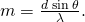Taking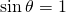and substituting the values offrom the preceding example gives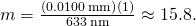Therefore, the largest integer m can be is 15, or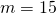.

Significance The number of fringes depends on the wavelength and slit separation. The number of fringes is very large for large slit separations. However, recall (see The Propagation of Light and the introduction for this chapter) that wave interference is only prominent when the wave interacts with objects that are not large compared to the wavelength. Therefore, if the slit separation and the sizes of the slits become much greater than the wavelength, the intensity pattern of light on the screen changes, so there are simply two bright lines cast by the slits, as expected, when light behaves like rays. We also note that the fringes get fainter farther away from the center. Consequently, not all 15 fringes may be observable.

Check Your Understanding In the system used in the preceding examples, at what angles are the first and the second bright fringes formed?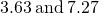, respectively

### Summary

• In double-slit diffraction, constructive interference occurs when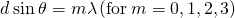, where d is the distance between the slits,is the angle relative to the incident direction, and m is the order of the interference.
• Destructive interference occurs when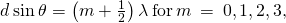.

### Conceptual Questions

Suppose you use the same double slit to perform Young’s double-slit experiment in air and then repeat the experiment in water. Do the angles to the same parts of the interference pattern get larger or smaller? Does the color of the light change? Explain.

Why is monochromatic light used in the double slit experiment? What would happen if white light were used?

Monochromatic sources produce fringes at angles according to. With white light, each constituent wavelength will produce fringes at its own set of angles, blending into the fringes of adjacent wavelengths. This results in rainbow patterns.

### Problems

At what angle is the first-order maximum for 450-nm wavelength blue light falling on double slits separated by 0.0500 mm?

Calculate the angle for the third-order maximum of 580-nm wavelength yellow light falling on double slits separated by 0.100 mm.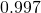What is the separation between two slits for which 610-nm orange light has its first maximum at an angle of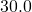?

Find the distance between two slits that produces the first minimum for 410-nm violet light at an angle of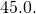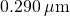Calculate the wavelength of light that has its third minimum at an angle ofwhen falling on double slits separated by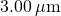. Explicitly show how you follow the steps from the Problem-Solving Strategy: Wave Optics, located at the end of the chapter.

What is the wavelength of light falling on double slits separated by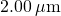if the third-order maximum is at an angle of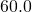?At what angle is the fourth-order maximum for the situation in the preceding problem?

What is the highest-order maximum for 400-nm light falling on double slits separated by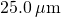?

62.5; since m must be an integer, the highest order is then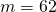.

Find the largest wavelength of light falling on double slits separated by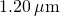for which there is a first-order maximum. Is this in the visible part of the spectrum?

What is the smallest separation between two slits that will produce a second-order maximum for 720-nm red light?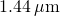(a) What is the smallest separation between two slits that will produce a second-order maximum for any visible light? (b) For all visible light?

(a) If the first-order maximum for monochromatic light falling on a double slit is at an angle of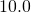, at what angle is the second-order maximum? (b) What is the angle of the first minimum? (c) What is the highest-order maximum possible here?

a.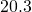; b.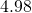; c. 5.76, the highest order is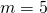.

Shown below is a double slit located a distance x from a screen, with the distance from the center of the screen given by y. When the distance d between the slits is relatively large, numerous bright spots appear, called fringes. Show that, for small angles (where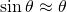, within radians), the distance between fringes is given by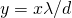Using the result of the preceding problem, (a) calculate the distance between fringes for 633-nm light falling on double slits separated by 0.0800 mm, located 3.00 m from a screen. (b) What would be the distance between fringes if the entire apparatus were submersed in water, whose index of refraction is 1.33?

a. 2.37 cm; b. 1.78 cm

Using the result of the problem two problems prior, find the wavelength of light that produces fringes 7.50 mm apart on a screen 2.00 m from double slits separated by 0.120 mm.

In a double-slit experiment, the fifth maximum is 2.8 cm from the central maximum on a screen that is 1.5 m away from the slits. If the slits are 0.15 mm apart, what is the wavelength of the light being used?

560 nm

The source in Young’s experiment emits at two wavelengths. On the viewing screen, the fourth maximum for one wavelength is located at the same spot as the fifth maximum for the other wavelength. What is the ratio of the two wavelengths?

If 500-nm and 650-nm light illuminates two slits that are separated by 0.50 mm, how far apart are the second-order maxima for these two wavelengths on a screen 2.0 m away?

1.2 mm

Red light of wavelength of 700 nm falls on a double slit separated by 400 nm. (a) At what angle is the first-order maximum in the diffraction pattern? (b) What is unreasonable about this result? (c) Which assumptions are unreasonable or inconsistent?

### Glossary

fringes
bright and dark patterns of interference
order
integer m used in the equations for constructive and destructive interference for a double slit